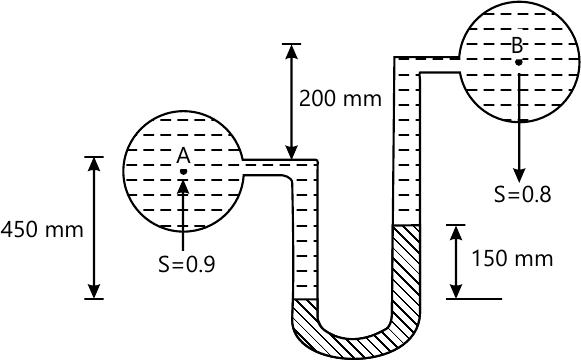VTU Mechanical Engineering (Semester 3)
Fluid Mechanics
June 2013
Total marks: --
Total time: --
INSTRUCTIONS
(1) Assume appropriate data and state your reasons
(2) Marks are given to the right of every question
(3) Draw neat diagrams wherever necessary

1 (a) Define the following propertise of fluid:
Specific weight
specific gravity
Vapour pressure
Capabilty
Surface tension
10 M
1 (b) A shaft of 250mm diameter rotates inside a sleve of 120mm long at 200rpm.If the thickness of oil film between shaft and sleeve is 1.5mm,find the power lost in friction.the dyanmic viocosity of oil used is 8 poise
10 M

2 (a) obtained the total pressure and the center of pressure on an inclined palin surface immersed in fluid
10 M
2 (b) A differential U -Tube mercury manometer is used to measure pressure between two water pipes A and B .Find the pressure at A if the level difference in mercury in the manommeter is 150mm as shown fig.the pressure at B is 300 kPa10 M

3 (a) Explain the method to find metacentric height experimently
8 M
3 (b) The stream function for a two -dimensional flow is given by φ=2xy.Find the velocity at the point p(4,2)also find the velocity potential function
12 M

4 (b) A 10km long water pipe is laid a slope of 3 in 4.The diameter of the lower end and upper end are 120mm and 180mm respectivly pressure gauges fixed at the lower end upper end reads 0.2MPa and respectively.Determine the flow rate of water through the pipe
10 M
4 (a) obtained the Eulers eqler equation of motion along a stream line and hence derive Bernuls equation for a stedy incompressible fluid flow
10 M

5 (a) Derive an expression for discharge through a rectangular notch
10 M
5(b) Using Buckingham's π-theorem prove that the frictional torque T of a disc of diameter D rotating at a speed N in fluid of viscosity μ and density ρ in a turbulent flow is given by $T=D^{5}N^{2}\rho \phi \left [ \frac{M}{D^{2}N\rho } \right ]$
10 M

6 (a) Derive Darcy 's equation for the loss of head due to friction in a circular pipe
10 M
6 (b) Water is supplied to a town having a popualtion of 1 lakh from a reservoir 6km away from the town and is stipulated that haif of the daily supply of 8-liters per head should be deliverd in 8hrs .What should b e the diameter of the supplier pipe?The loss of head due to friction in the line is 2m.Take f=0.002
10 M

7 (a) Derive Hagen -poiseuille's equation for viscows flow through a circular pipe
10 M
7 (b) A pipe of daimeter 240mm and lenghth is laid at a slope of 1 in 250 .An oil of specification gravity 0.85 and viscosity 180cp is pumped up at a rate of 10liters /sec. find the head lost due to friction and the power required to pump the oil
10 M

8 (a) Explain the following:
Drag
Lift
Momentum thickness
Mach number
mach cone
10 M
8 (b) A flat plate 2m × 2m moves at 40km/hour in the stationay air density 1.2kg/m3If the coefficient of drag and lift are 0.75 respectivly,determine
the lift force
the drag force
the resultant force
The power required to keep the plate in motion
10 M

More question papers from Fluid Mechanics